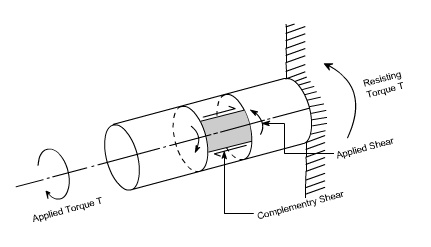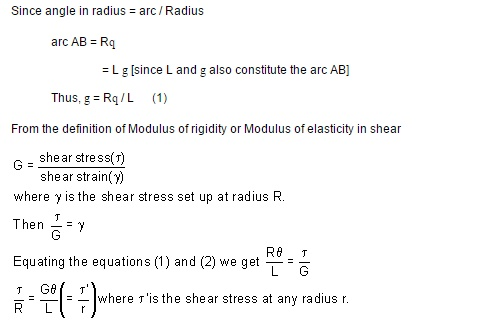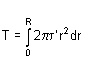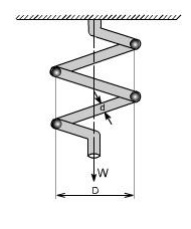Home | | Strength of Materials for Mechanical Engineers | Stepped shaft ,Twist and torsion stiffness

# Stepped shaft ,Twist and torsion stiffness

Shaft: The shafts are the machine elements which are used to transmit power in machines.

Stepped shaft ,Twist and torsion stiffness

–Compound shafts –Fixed and simply supported shafts

Shaft: The shafts are the machine elements which are used to transmit power in machines.

Twisting Moment: The twisting moment for any section along the bar / shaft is defined to be the algebraic sum of the moments of the applied couples that lie to one side of the section under consideration. The choice of the side in any case is of course arbitrary.

Shearing Strain: If a generator a ?? b is marked on the surface of the unloaded bar, then after the twisting moment 'T' has been applied this line moves to ab'. The angle ???'

measured in radians, between the final and original positions of the generators is defined as the shearing strain at the surface of the bar or shaft. The same definition will hold at any interior point of the bar.Modulus of Elasticity in shear: The ratio of the shear stress to the shear strain is called the modulus of elasticity in shear OR Modulus of Rigidity and in represented by the symbolAngle of Twist: If a shaft of length L is subjected to a constant twisting moment T along its length, than the angle ? through which one end of the bar will twist relative to the other is known is the angle of twist.Despite the differences in the forms of loading, we see that there are number of similarities between bending and torsion, including for example, a linear variation of stresses and strain with position.

In torsion the members are subjected to moments (couples) in planes normal to their axes.

For the purpose of desiging a circular shaft to withstand a given torque, we must develop an equation giving the relation between twisting moment, maximum shear stress produced, and a quantity representing the size and shape of the cross-sectional area of the shaft.

Not all torsion problems, involve rotating machinery, however, for example some types of vehicle suspension system employ torsional springs. Indeed, even coil springs are really curved members in torsion as shown in figure.Many torque carrying engineering members are cylindrical in shape. Examples are drive shafts, bolts and screw drivers.

Simple Torsion Theory or Development of Torsion Formula : Here we are basically interested to derive an equation between the relevant parameters

Relationship in Torsion:1 st Term: It refers to applied loading ad a property of section, which in the instance is the polar second moment of area.

2 nd Term: This refers to stress, and the stress increases as the distance from the axis increases.

3 rd Term: it refers to the deformation and contains the terms modulus of rigidity & combined term ( ??? l) which is equivalent to strain for the purpose of designing a circular shaft to with stand a given torque we must develop an equation giving the relation between Twisting moments max m shear stain produced and a quantity representing the size and shape of the cross ??sectional area of the shaft.Refer to the figure shown above where a uniform circular shaft is subjected to a torque it can be shown that every section of the shaft is subjected to a state of pure shear, the moment of resistance developed by the shear stresses being every here equal to the magnitude, and opposite in sense, to the applied torque. For the purpose of deriving a simple theory to describe the behavior of shafts subjected to torque it is necessary make the following base assumptions.

Assumption:

(i)                The materiel is homogenous i.e of uniform elastic properties exists throughout the material.

(ii) The material is elastic, follows Hook's law, with shear stress proportional to shear strain.

(iii) The stress does not exceed the elastic limit.

(iv)               The circular section remains circular

(v)Cross section remain plane.

(vi)               Cross section rotate as if rigid i.e. every diameter rotates through the same angle.Consider now the solid circular shaft of radius R subjected to a torque T at one end, the other end being fixed Under the action of this torque a radial line at the free end of the shaft twists through an angle , point A moves to B, and AB subtends an angle ' at the fixed end. This is then the angle of distortion of the shaft i.e the shear strain.arc AB = R?

= L ? [since L and ? also constitute the arc AB]

Thus, ? = R? / L (1)

From the definition of Modulus of rigidity or Modulus of elasticity in shear

Stresses: Let us consider a small strip of radius r and thickness dr which is subjected to shear stress t'.The force set up on each element

= stress x area

= t' . 2 p r dr . r

== 2 p t' . r2. dr

The total torque T on the section, will be the sum of all the contributions.Since t' is a function of r, because it varies with radius so writing down t' in terms of r from the equation (1).

This force will produce a moment or torque about the center axis of the shaft.The total torque T on the section, will be the sum of all the contributions.

Since ?' is a function of r, because it varies with radius so writing down??' in terms of r from the equation (1).

Where

T = applied external Torque, which is constant over Length L;

J = Polar moment of Inertia[ D = Outside diameter ; d = inside diameter ]

G = Modules of rigidity (or Modulus of elasticity in shear)

q = It is the angle of twist in radians on a length L.

Tensional Stiffness: The tensional stiffness k is defined as the torque per radius twist

i.e, k = T /q= GJ / L

Problem 1

A stepped solid circular shaft is built in at its ends and subjected to an externally applied torque. T0 at the shoulder as shown in the figure. Determine the angle of rotation ?0 of the shoulder section where T0 is applied ?Solution: This is a statically indeterminate system because the shaft is built in at both ends. All that we can find from the statics is that the sum of two reactive torque TA and TB at the built ?? in ends of the shafts must be equal to the applied torque T0

Thus TA+ TB = T0 ------         (1)

[from static principles]

Where TA ,TB are the reactive torque at the built in ends A and B. wheeras T0 is the applied torque

From consideration of consistent deformation, we see that the angle of twist in each portion of the shaft must be same.

i.e qa = q b = q 0

using the relation for angle of twistN.B: Assuming modulus of rigidity G to be same for the two portions

So the defines the ratio of TA and TB

So by solving (1) & (2) we getNon Uniform Torsion: The pure torsion refers to a torsion of a prismatic bar subjected to torques acting only at the ends. While the non uniform torsion differs from pure torsion in a sense that the bar / shaft need not to be prismatic and the applied torques may vary along the length.Here the shaft is made up of two different segments of different diameters and having torques applied at several cross sections. Each region of the bar between the applied loads between changes in cross section is in pure torsion, hence the formula's derived earlier may be applied. Then form the internal torque, maximum shear stress and angle of rotation for each region can be calculated from the relationThe total angle to twist of one end of the bar with respect to the other is obtained by summation using the formulaIf either the torque or the cross section changes continuously along the axis of the bar, then the ? (summation can be replaced by an integral sign ( ? ). i.e We will have to consider a differential element.After considering the differential element, we can writeSubstituting the expressions for Tx and Jx at a distance x from the end of the bar, and then integrating between the limits 0 to L, find the value of angle of twist may be determined.Application to close-coiled helical springs

Closed Coiled helical springs subjected to axial loads:

Definition: A spring may be defined as an elastic member whose primary function is to deflect or distor under the action of applied load; it recovers its original shape when load is released.

or

Springs are energy absorbing units whose function is to store energy and to restore it slowly or rapidl depending on the particular application.

Important types of springs are:

There are various types of springs such as

(i) helical spring: They are made of wire coiled into a helical form, the load being applied along the axi of the helix. In these type of springs the major stresses is torsional shear stress due to twisting. They are both used in tension and compression.(ii) Spiral springs: They are made of flat strip of metal wound in the form of spiral and loaded in torsion.

In this the major stresses are tensile and compression due to bending.(iv) Leaf springs: They are composed of flat bars of varying lengths clamped together so as to obtai greater efficiency . Leaf springs may be full elliptic, semi elliptic or cantilever types, In these type o springs the major stresses which come into picture are tensile & compressive.These type of springs are used in the automobile suspension system.

Uses of springs :

(a)To apply forces and to control motions as in brakes and clutches.

(b) To measure forces as in spring balance.

(c) To store energy as in clock springs.

(d) To reduce the effect of shock or impact loading as in carriage springs.

(e) To change the vibrating characteristics of a member as inflexible mounting of motors.

Derivation of the Formula :

In order to derive a necessary formula which governs the behaviour of springs, consider a closed coile spring subjected to an axial load W.Let

D = mean coil diameter

d = diameter of spring wire n = number of active coils

C = spring index = D / d For circular wires l = length of spring wire

G = modulus of rigidity x = deflection of spring q = Angle of twist

when the spring is being subjected to an axial load to the wire of the spring gets be twisted like a shaft.

If q is the total angle of twist along the wire and x is the deflection of spring under the action of load W along the axis of the coil, so that

x = D / 2 .

again l =   D n [ consider ,one half turn of a close coiled helical spring ]Maximum shear stress in spring section including Wahl Factor

Wahl's factor

Assumptions: (1) The Bending & shear effects may be neglected

(2) For the purpose of derivation of formula, the helix angle is considered to be so small that it may be neglected.

Any one coil of a such a spring will be assumed to lie in a plane which is nearly r to the axis of the spring. This requires that adjoining coils be close together. With this limitation, a section taken perpendicular to the axis the spring rod becomes nearly vertical. Hence to maintain equilibrium of a segment of the spring, only a shearing force V = F and Torque T = F. r are required at any X –section. In the analysis of springs it is customary to assume that the shearing stresses caused by the direct shear force is uniformly distributed and is negligible

so applying the torsion formula.

Using the torsion formula i.eStudy Material, Lecturing Notes, Assignment, Reference, Wiki description explanation, brief detail
Mechanical : Strength of Materials : Torsion : Stepped shaft ,Twist and torsion stiffness |